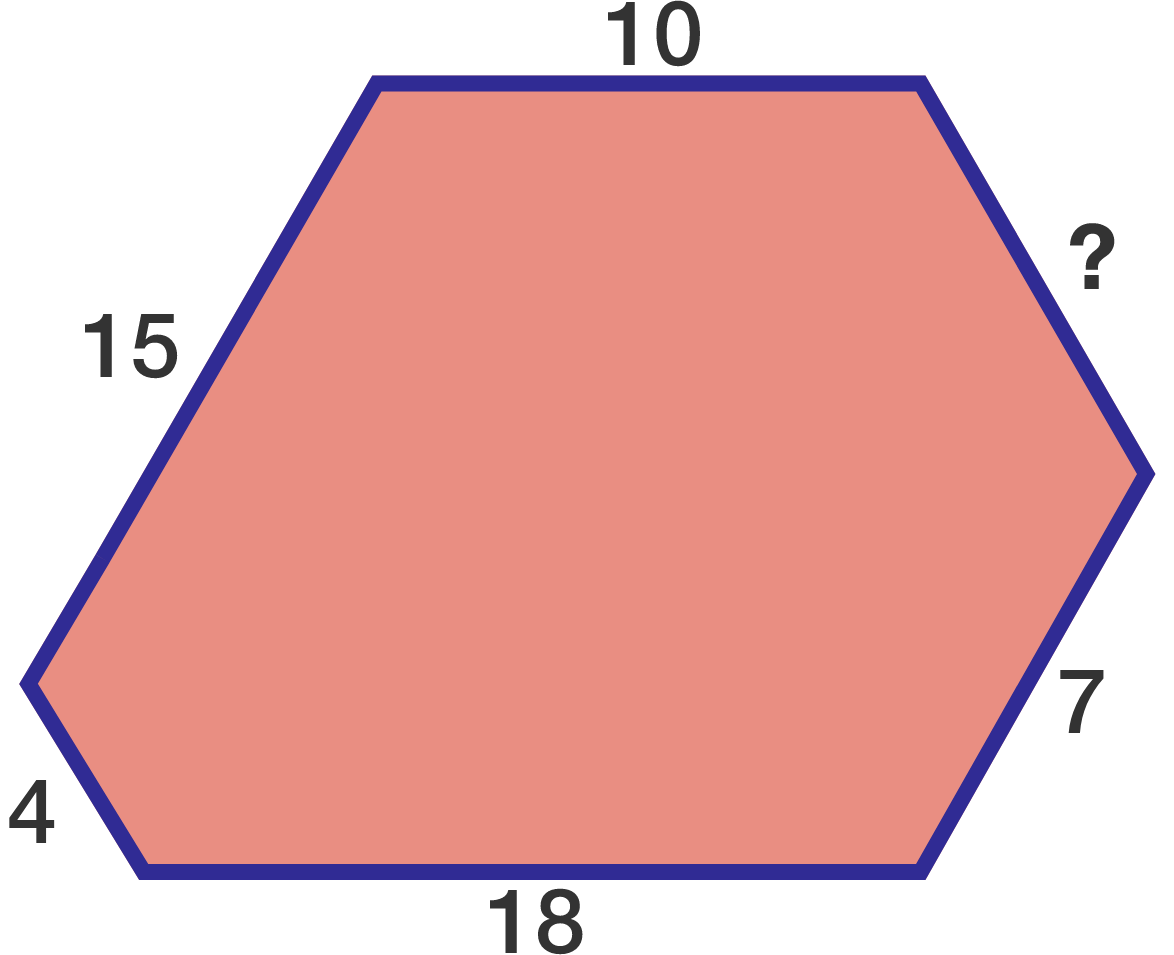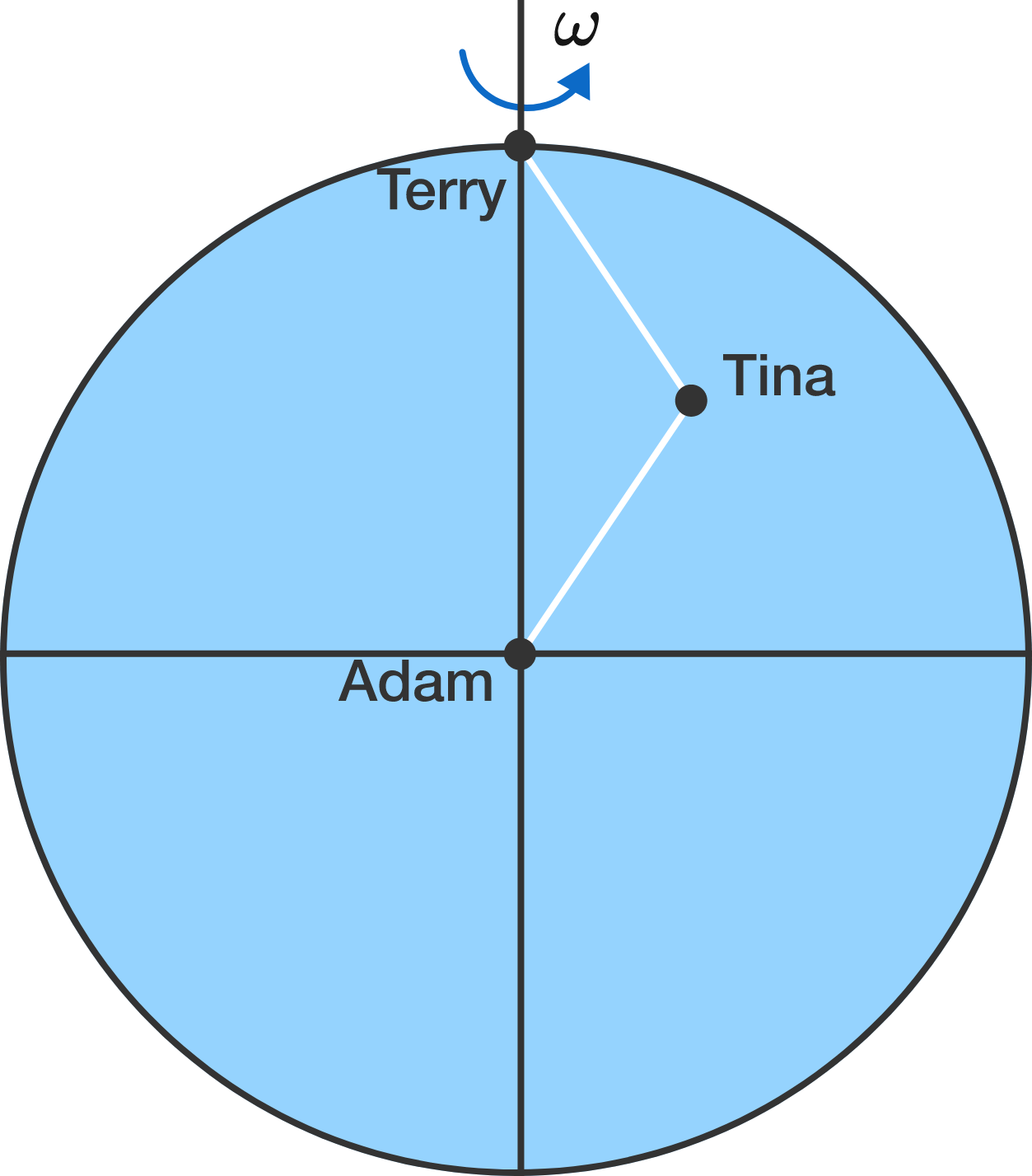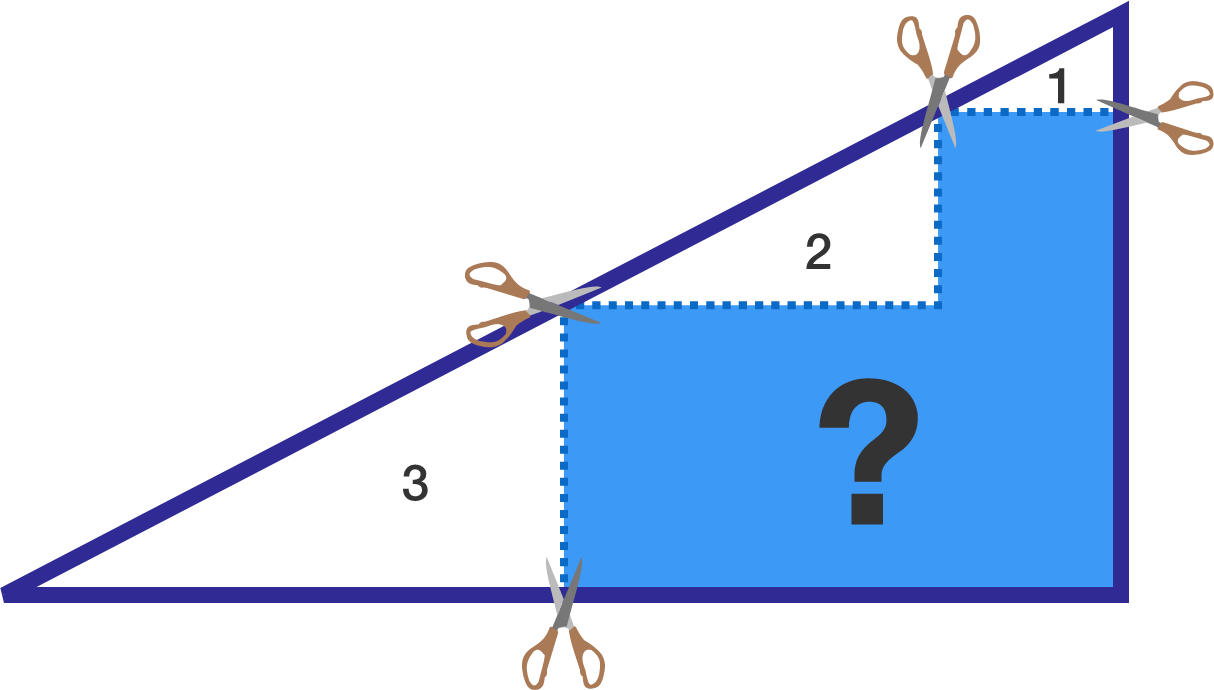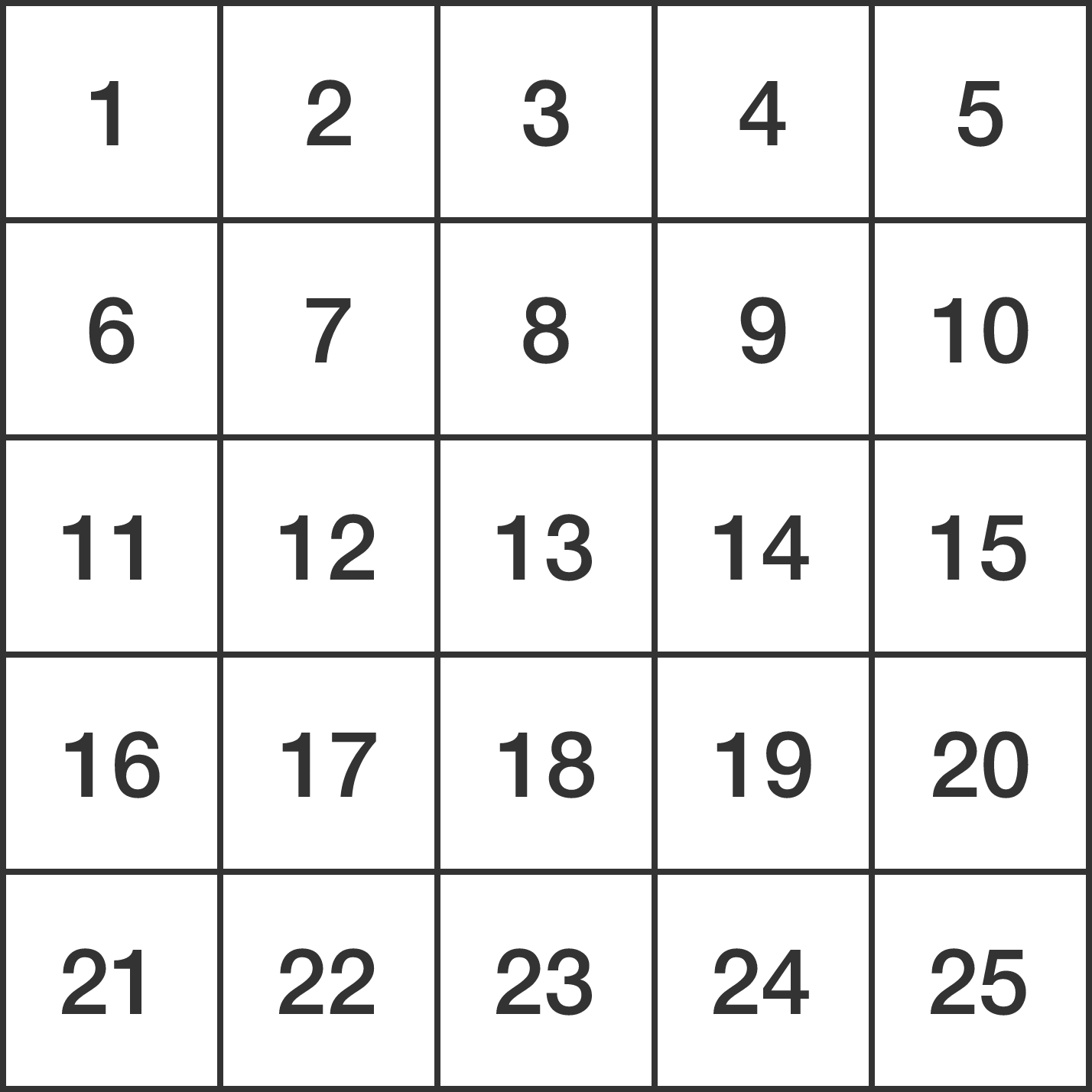# Problems of the Week

Contribute a problem

# 2017-07-03 Intermediate

The hexagon below has interior angles that measure all the same. As shown, only five of the six side lengths are known: 10, 15, 4, 18, 7. What is the unknown side length?Two generals, Adam and Terry, aim their long range cannons at a third general Tina. Each general calibrates the explosive power of their cannon so it will hit Tina's base using the least energy possible. Tina's base is an equal distance (along the surface of the Earth Earth) from Adam and Terry.

If the positions of Adam and Terry are as shown above, which general will need to launch their cannon rounds at a smaller speed in order to hit Tina?

Assumptions and Details

• The Earth is perfectly spherical.
• The launch speed of either cannon is high enough that the atmosphere is negligible.

From the large right triangle, three smaller right triangles (in white) of respective areas 1, 2, 3 are cut out. Find the area of the remaining figure (in blue), to 2 decimal places.Let $f$ be a polynomial function with integer coefficients such that $f(2017) = 1.$ Which of the following can equal $f(2020)?$

Filling a $5 \times 5$ grid with distinct integers from 1 to 25, what is the minimum of the maximum of the differences between 2 squares that share a common side?


Hint: As an explicit example, for the naive arrangement belowthe maximum of the differences between 2 squares sharing a common side is equal to 5. This is because, in the above table which is one of all $25!$ possible arrangements, the maximum never exceeds 5, as can be seen by the differences between many neighboring cells: $6-1=7-2=\cdots=24-19=25-20,$ which are all equal to 5. Hence, the minimum of the maximum we are looking for is at most 5.

×